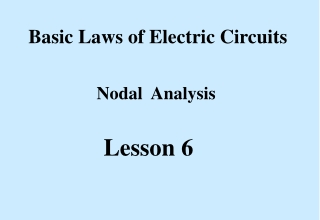DownloadDownload PresentationLesson 6

Lesson 6

Télécharger la présentationLesson 6

- - - - - - - - - - - - - - - - - - - - - - - - - - - E N D - - - - - - - - - - - - - - - - - - - - - - - - - - -
Presentation Transcript

1. Basic Laws of Electric Circuits Nodal Analysis Lesson 6

2. Basic Circuits Nodal Analysis: The Concept. • Every circuit has n nodes with one of the nodes being • designated as a reference node. • We designate the remaining n – 1 nodes as voltage nodes • and give each node a unique name, vi. • At each node we write Kirchhoff’s current law in terms • of the node voltages. 1

3. Basic Circuits Nodal Analysis: The Concept. • We form n-1 linear equations at the n-1 nodes • in terms of the node voltages. • We solve the n-1 equations for the n-1 node voltages. • From the node voltages we can calculate any branch • current or any voltage across any element. 2

4. Basic Circuits Nodal Analysis: Concept Illustration: Figure 6.1: Partial circuit used to illustrate nodal analysis. Eq 6.1 3

5. Basic Circuits Nodal Analysis: Concept Illustration: Clearing the previous equation gives, Eq 6.2 We would need two additional equations, from the remaining circuit, in order to solve for V1, V2, and V3 4

6. Basic Circuits Nodal Analysis: Example 6.1 Given the following circuit. Set-up the equations to solve for V1 and V2. Also solve for the voltage V6. Figure 6.2: Circuit for Example 6.1. 5

7. Basic Circuits Nodal Analysis: Example 6.1, the nodal equations. Eq 6.3 Eq 6.4 6

8. Basic Circuits Nodal Analysis: Example 6.1: Set up for solution. Eq 6.3 Eq 6.4 Eq 6.5 Eq 6.6 7

9. Basic Circuits Nodal Analysis: Example 6.2, using circuit values. Figure 6.3: Circuit for Example 6.2. Find V1 and V2. At v1: Eq 6.7 At v2: Eq 6.8 8

10. Basic Circuits Nodal Analysis: Example 6.2: Clearing Equations; From Eq 6.7: V1 + 2V1 – 2V2 = 20 or Eq 6.9 3V1 – 2V2 = 20 From Eq 6.8: 4V2 – 4V1 + V2 = -120 or -4V1 + 5V2 = -120 Eq 6.10 Solution: V1 = -20 V, V2 = -40 V 9

11. Basic Circuits Nodal Analysis: Example 6.3: With voltage source. Figure 6.4: Circuit for Example 6.3. At V1: Eq 6.11 At V2: Eq 6.12 10

12. Basic Circuits Nodal Analysis: Example 6.3: Continued. Collecting terms in Equations (6.11) and (6.12) gives Eq 6.13 Eq 6.14 11

13. Basic Circuits Nodal Analysis: Example 6.4: Numerical example with voltage source. Figure 6.5: Circuit for Example 6.4. What do we do first? 12

14. Basic Circuits Nodal Analysis: Example 6.4: Continued At v1: Eq 6.15 At v2: Eq 6.16 13

15. Basic Circuits Nodal Analysis: Example 6.4: Continued Clearing Eq 6.15 4V1 + 10V1 + 100 – 10V2 = -200 or 14V1 – 10V2 = -300 Eq 6.17 Clearing Eq 6.16 4V2 + 6V2 – 60 – 6V1 = 0 or -6V1 + 10V2 = 60 Eq 6.18 V1 = -30 V, V2 = -12 V, I1 = -2 A 14

16. Basic Circuits Nodal Analysis: Example 6.5: Voltage super node. Given the following circuit. Solve for the indicated nodal voltages. super node Figure 6.6: Circuit for Example 6.5. When a voltage source appears between two nodes, an easy way to handle this is to form a super node. The super node encircles the voltage source and the tips of the branches connected to the nodes. 15

17. Basic Circuits Nodal Analysis: Example 6.5: Continued. Constraint Equation V2 – V3 = -10 Eq 6.19 At V1 Eq 6.20 At super node Eq 6.21 16

18. Basic Circuits Nodal Analysis: Example 6.5: Continued. Clearing Eq 6.19, 6.20, and 6.21: Eq 6.22 7V1 – 2V2 – 5V3 = 60 -14V1 + 9V2 + 12V3 = 0 Eq 6.23 Eq 6.24 V2 – V3 = -10 Solving gives: V1 = 30 V, V2 = 14.29 V, V3 = 24.29 V 17

19. Basic Circuits Nodal Analysis: Example 6.6: With Dependent Sources. Consider the circuit below. We desire to solve for the node voltages V1 and V2. Figure 6.7: Circuit for Example 6.6. In this case we have a dependent source, 5Vx, that must be reckoned with. Actually, there is a constraint equation of Eq 6.25 18

20. Basic Circuits Nodal Analysis: Example 6.6: With Dependent Sources. At node V1 At node V2 The constraint equation: 19

21. Basic Circuits Nodal Analysis: Example 6.6: With Dependent Sources. Clearing the previous equations and substituting the constraint VX = V1 - V2 gives, Eq 6.26 Eq 6.27 which yields, 20

22. circuits End of Lesson 6 Nodal Analysis